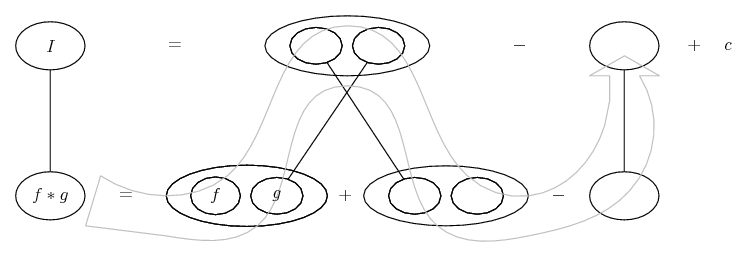# Thread: Integration by parts

1. ## Integration by parts

Hey, got a revision sheet question I cant seem to get right heres what I have:

between limits 1 and 0 find the integral of: 3x^2.e^x/2.dx

my working:

u.v-integral v.du/dx

e^x/2.x^3 - integral x^3.1/2e^x/2

=(e^x/2.x^3 - 1/4x^4.e^x/2) between 1 and 0

= 1.237

but the correct answer is down as 15.78

any help would be appreciated

2. You're not assigning the right parts of the integrand to u and dv. Use ILATE. (I prefer ILATE to the LIATE rule in the wiki article.) The power of x should be going down as you integrate.

3. ok thanks i done this originally but the answer it gives me is miles off,

when i integrate e^x/2 is it right that it just leaves 2e^x/2 ?

4. Yes, that's right.

Just in case a picture helps...... where (key in spoiler) ...

Spoiler:... is the product rule. Straight continuous lines differentiate downwards (integrate up) with respect to x. And,... is lazy integration by parts, doing without u and v.

So it's "integration by parts twice" in order to keep the whole of the bottom row equal to the product you start with (and your integral is the top row).
_________________________________________

Don't integrate - balloontegrate!

Balloon Calculus; standard integrals, derivatives and methods

Balloon Calculus Drawing with LaTeX and Asymptote!

5. Alternatively, you can use tabular integration. It's probably the quickest way (assuming you do it right).

#### Search Tags

integration, parts